# Markov spectrum problem

(diff) ← Older revision | Latest revision (diff) | Newer revision → (diff)

A problem in number theory which arises in connection with the distribution of the normalized values of arithmetic minima of indefinite binary quadratic forms (cf. Binary quadratic form). Let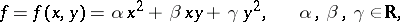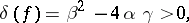and let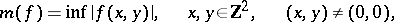be the uniform arithmetic minimum of the form. The number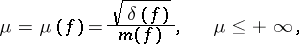is called the Markov constant of. The set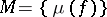, whereruns through all real indefinite quadratic forms, is called the Markov spectrum. The Markov constant and the Markov spectrum have been defined in various ways; in particular, A.A. Markov in

considered the set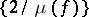. It is known that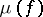is an invariant of a rayof classes of forms, that is, of a set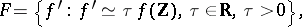(1)

since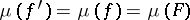. Each ray of classesis in one-to-one correspondence with a doubly-infinite (infinite in both directions) sequence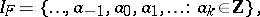such that if one puts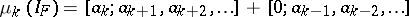(is the notation for a continued fraction), then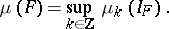The Markov problem can be stated as follows: 1) describe the Markov spectrum; and 2) for each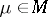, describe the set of forms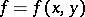(or the rays) for which. The problem was solved by Markov for the initial part of the spectrumdefined by the condition. This part of the spectrum is a discrete set: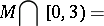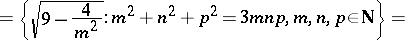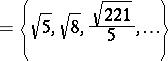with the unique limit point 3 (a condensation point of);,andrun through all positive integer solutions of Markov's Diophantine equation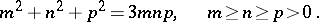(2)

In this case there corresponds to each point of this part of the spectrum precisely one ray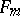, given by a Markov form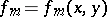, with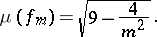A solution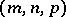of (2) is called a Markov triple; the numberis called a Markov number. The Markov formis associated to the Markov number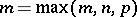as follows. Letbe defined by the conditions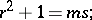then, by definition,The setis closed and there is a smallest number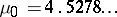such that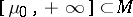and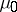borders the interval of contiguity of.

The Markov problem is closely related to the Lagrange–Hurwitz problem on rational approximation of a real number. The quantity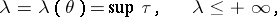where the least upper bound is taken over all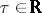,, for which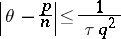has an infinite set of solutions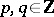,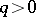, is called a Lagrange constant. The set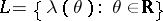is called the Lagrange spectrum. It is natural to regard Lagrange's theorem as the first result in the theory of the Lagrange spectrum: All convergents of the continued fraction expansion ofsatisfyIf, that is, if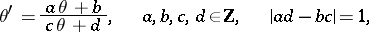then, where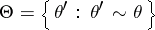is an equivalence class of numbers. Ifis expanded as a continued fraction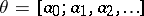, then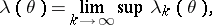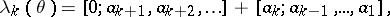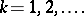Thus, the Lagrange–Hurwitz problem can be stated as: a) describe the Lagrange spectrum; and b) for each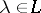, describe the set of numbers(or classes) for which.

Forthis problem reduces to the Markov problem; moreover,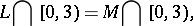and to each,, corresponds precisely one class, described by the Markov form, provided the unicity conjecture is true. It has been proved that, like, is a closed set; that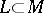but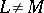; that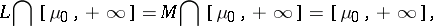whereborders the interval of contiguity of. Research into the structure ofand the connection betweenandis described in . For generalizations and analogues of the Markov spectrum problem and "isolation phenomena" see , , .

How to Cite This Entry:
Markov spectrum problem. Encyclopedia of Mathematics. URL: http://encyclopediaofmath.org/index.php?title=Markov_spectrum_problem&oldid=13629
This article was adapted from an original article by A.V. Malyshev (originator), which appeared in Encyclopedia of Mathematics - ISBN 1402006098. See original article